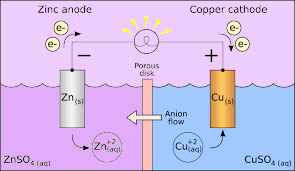# Cathode and Anode

Before we learn about cathode and anode we need to first understand what an electrode is. As per the general definition, an electrode is a substance that helps in the conduction of electricity wherein the electric current either enters or leaves the non-metallic medium like an electrolytic cell.

In simple terms, an electrode is a conductor that helps in establishing electrical contact with a non-metallic part of the circuit. Electrodes consist of two main points known as cathode and anode which basically describe the direction of flow of current.

## What are Cathode and Anode?

Let us understand what cathode and anode exactly mean. They are both defined by the flow of current. Therefore, a cathode is an electrode from which the current exits a polarized electrical device. Likewise, an anode is the electrode from which a current enters into a polarized electrical device.

The terms were finalized in 1834 by William Whewell who adapted the words from the Greek word (kathodos), ‘descent’ or ‘way down’. William had consulted with Michael Faraday for the coining of the terms.Cathode and Anode Example

### Cathode

When we talk about cathode in chemistry, it is said to be the electrode where reduction occurs. This is common in an electrochemical cell. Here, the cathode is negative as the electrical energy that is supplied to the cell results in the decomposition of chemical compounds. However, it can also be positive as in the case of a galvanic cell where a chemical reaction leads to the generation of electrical energy.

In addition, a cathode is said to be either hot cathode or a cold cathode. A cathode which is heated in the presence of a filament to emit electrons by thermionic emission is known as a hot cathode whereas cold cathodes are not heated by any filament. A cathode is usually flagged as “cold” if it emits more electrons compared to the ones generated by thermionic emission alone.

### Anode

In the most basic form, an anode in electrochemistry is the point where an oxidation reaction occurs. Generally, at an anode, negative ions or anions due to its electrical potential tend to react and give off electrons. These electrons then move up and into the driving circuit.

If we take a galvanic cell, the anode is negative in nature and the electrons mostly move towards the external part of the circuit. In an electrolytic cell, it is again positive. Additionally, an anode can be a plate or wire having an excess positive charge.

## Difference Between Anode And Cathode

Here are some of the key differences between cathode and anode.

 Anode Cathode The anode is the electrode where electricity moves into. The cathode is the electrode where electricity is given out or flows out of. The anode is usually the positive side. A cathode is a negative side. It acts as an electron donor. It acts as an electron acceptor. In an electrolytic cell, oxidation reaction takes place at the anode. In an electrolytic cell, a reduction reaction takes place at the cathode. In galvanic cells, an anode can become a cathode. In galvanic cells, a cathode can become an anode.

1. What is the charge of an anode and cathode?

Ans: The anode is regarded as negative in a galvanic (voltaic) cell and the cathode is deemed positive. This seems appropriate because the anode is the origin of electrons and where the electrons flow is the cathode.

2. Does oxidation occur at the anode or cathode?

Ans: The anode is where the response to oxidation occurs. That’s where the metal loses electrons, in other words.

3. What is the charge on anode and cathode?

Ans: There is an oxidation response at the anode. The oxidized species would lose electrons, leaving this electrode with an accumulation of electrons. Therefore, the anode is charged negatively. In contrast to the cathode, there is a reduction response where the decreased species would obtain electrons. Therefore, the electrode, i.e. the cathode, lacks electrons and is therefore charged positively.

4. Are cations positive or negative?

Ans: A cation is defined as a positively charged ion or an atom that has lost an electron.

5. What are the materials used for anode and cathode?

Ans: Metals like zinc and lithium are often used as substrates for anodes.

6. What is anode and cathode in corrosion?

Ans: Iron metal functions as the anode in a galvanic cell during the corrosion phase and is oxidized to Fe2+; at the cathode, oxygen is decreased to water.

7. Does reduction always occur at the cathode?

Ans: Reduction at the cathode always happens, and oxidation at the anode always happens. Because decrease is the addition of electrons, electrons have to move to the reduction site.

8. Is LED cathode positive or negative?

Ans: LEDs are generally labelled in some way by their cathode. The cathode should be linked to the driving voltage source’s floor or adverse side and the anode to the positive side.